Reach Us+44-1904-929220
An Experimental and Analysis Study on Vibration Control Devices Called as Hybrid Scaling - Frame | OMICS International
Journal of Civil & Environmental Engineering
All submissions of the EM system will be redirected to Online Manuscript Submission System. Authors are requested to submit articles directly to Online Manuscript Submission System of respective journal.

# An Experimental and Analysis Study on Vibration Control Devices Called as Hybrid Scaling - Frame

Hideaki Azu1*, Akinobu Kano1, Takumi Ito1, Donghang Wu2, Takashi Nagumo3 and Haruhiko Hirata3

1Department of Architecture, Tokyo University of Science, Japan

2Wu Office, P.R. China

3Hory Corporations, Japan

*Corresponding Author:
Hideaki Azu
Department of Architecture Tokyo
University of Science, Japan
Tel:
+81 3-3260-4271
E-mail:
[email protected]

Received date: July 06, 2016; Accepted date: July 13, 2016; Published date: July 15, 2016

Citation: Azu H, Kano A, Ito T, Wu D, Nagumo T, et al. (2016) An Experimental and Analysis Study on Vibration Control Devices Called as Hybrid Scaling - Frame. J Civil Environ Eng S3:001. doi:10.4172/2165-784X.S3-001

Copyright: © 2016 Mbamalu ON, et al. This is an open-access article distributed under the terms of the Creative Commons Attribution License, which permits unrestricted use, distribution, and reproduction in any medium, provided the original author and source are credited.

Visit for more related articles at Journal of Civil & Environmental Engineering

#### Abstract

The innovated vibration control device called as “Scaling Frame” (abbreviated as “SF”) structure is proposed by the author. SF structure consists of beam-column frame, diagonal bracing, and SF device (abbreviated as “SFD”) made of Aluminum or Steel. And, vibration energy is absorbed by plastic behavior of diagonal deformation of SFD. SF structure has been already adopted for low-rise wooden buildings in Japan. In this study, SF structure is assumed to apply on multi-story steel frames, that is, in which high strength and rigidity are required. So then, a hybrid SFD (abbreviated as “HSFD”) which consist of various shapes of SFD is developed herein. And also, to clarify the fundamental restoring force characteristics of HSFD, horizontal static cyclic loading test is conducted. From tests results, it is confirmed that the rigidity and strength of devices is expressed in the sum of each SFD which constitutes HSFD, and the stable hysteresis behavior is presented. It means that the strength and rigidity can be adjusted easily by combination of SFD. Furthermore, the analytical study is done by use of restoring force characteristics model of each shapes of SFD. From the comparison between test results and analysis results, it is observed that the proposed analytical model of HSFD can pursue the test result during cyclic loading well. And also, by use of various type of SFD in substitution for normal type of SFD, it is possible to decrease the number of necessary device more.

#### Keywords

Horizontal loading test; Restoring force characteristics model; Steel frame structure; Scaling-Frame; Vibration control device

#### Introduction

Recently, a various type of vibration control device has been developed, and most devices are adapted to actual building structure. But they have some problems of durability and stability velocity. And, they consist of special device and material, so there are some difficulties of getting material and construction technics. In contrast, it is expected that SF structure can solve these difficulties. SFD is made of steel or aluminum, that is, SFD has some advantage such as workability, productivity and containment. SF structure consists of beam-column frame, diagonal bracing, and SFD as shown in Figure 1, and vibration energy is absorbed by plastic behavior of diagonal deformation of SFD. SF structure has been used in wooden house already. So in this study, to apply SFD on steel structures is intended. Table 1 explains the developing plan about SF structure. In this study, to apply SFD on multi-story steel frames in which high strength and rigidity are required, HSFD which consist of various shapes of SFD is developed. And, to investigate the global restoring force characteristics of HSFD, the horizontal static cyclic loading test was conducted. And also, the analytical study was done by use of restoring force characteristics model of HSFD.

Target SFD (basic type) HSFD
Wood Steel Steel
Material A S A S and A
Test 1 1* 1* 2
Analysis - 1* 1* 2
Design 1 3 3 3

Table 1: Developing plan about SF structure.

#### General Description of SF Structure

Herein, the mechanical and geometrical property of SF structure is explained. As a definition of SF structure, the relation of size of outframes and SFD is defined as reduction rate “α” as shown in Figure 1. Figure 2 shows the resistant mechanism of SFD. From this model, the rigidity KSF and yield strength Py of basic type of SFD are obtained theoretically by using α as follows: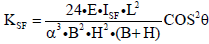(1)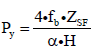(2)

E: The young’s modulus of the SFD

ISF: Moment of inertia of area of the SFD

ZSF: Elastic modulus of SFD B: the length of the beam H: the length of the column

L: The diagonal length of beam-column frame

θ: The angle between frame and diagonal member

fb: Allowable bending stress of the SFD.

From the above equations, it can be said that KSF is inverse proportion to a cube of reduction rate α, and the strength is inverse proportion to reduction rate α. In other words, the smaller the reduction rate of SFD, the larger the rigidity and the strength, and high energy absorption can be expected.

Herein, when shear deformation of rectangular frame progresses, it is clarified geometrically that the diagonal displacement of compressive grows larger than the one of extension. This property is applied to SF structure as shown in Figure 3. Especially, in the diagonal deformation of the SFD, this property appears more conspicuously. Thus, the compressive deformation of the diagonal bracing on compressive side is small, and diagonal axial force will be reduced in large deformation range as shown in Figure 4. So the lateral buckling of diagonal member on compressive side is prevented. On the other hands, the diagonal axial force on tensile side will resist increasingly with progress of the deformation as shown in Figure 4. So bending reflection of the SF is restricted in large deformation range, and rigidity in the axial direction of the SFD becomes dominant.

#### Summary of Horizontal Static Loading Test

General description of loading test

This paper studies the fundamental restoring force characteristics of HSFD experimentally. Herein, to investigate them, horizontal static loading test was conducted. Table 2 shows name of two types of SFD which constitutes HSFD. Each types of SFD is made of steel (SS400) or aluminum (A1050). Table 3 shows the mechanical properties of the steel and aluminum used for the test specimen. Herein, σy is yield strength and σu is tensile strength. εy is yield strain and εp is the strain when strain hardening begin. And, E is Young’s modulus. SS400 and A1050 present the grade of JIS (Japanese Industrial Standards) standard. Figure 5 shows the test specimen of the HSFD. Herein, HSFD is consisting of two shapes of SFD.

Member Shape Name α
[%]
σy
[N/mm2]
σu
[N/mm2]
εy
[μ]
εp
[μ]
E
[N/mm2]
JIS
Grade
HSFD Double type S-Double 4, 9 352 445 1667 13228* 205000 SS400
Cross type A-Cross 8.75 52* 78* 765* 6072* 68000 A1050
bracing - - - 359 428 - - - STK400

Table 2: Name of test specimens and technical properties of member.

SFD α Thickness Number
[%] [mm]
Basic type 9 113 124
Double type 12, 8 115 68
HSFD D*1 13, 9.5 113 73 68
C*2 12.5 40

Table 3: Size of SFD and the number of dumper.

Test set up and loading test methods

Figure 6 shows a test set up. The SFD is connected with column jig through gusset plate and diagonal bracing. Columns are supported to the beam by pin joint. The horizontal force is measured from the load cell built in to the loading jack. The horizontal displacement at the top of the column is measured by using a rod type displacement transducer. The strain gauges are placed on the each SFD and the diagonal bracing as shown in Figure 5. Loading pattern is cyclic. The loading program is arranged by the reference of the target displacement of the beam. The maximum angles of the column at each loop on the cyclic tests are 1/300, 1/200, 1/100, 1/75 and 1/50 rad herein, the loop of the 1/300, 1/200, 1/100, and 1/75 rad are repeated two times. In addition, the loop of the 1/50 rad is repeated five times to investigate ultimate state of HSFD.

#### Results of Test Study

Figure 7 shows the relation of horizontal load and horizontal displacement of test result. And, Figure 8 shows the skeleton curve of test result. Herein, Figure 8 shows the test result to the second loop at the drift angle of 1/50 rad, because some little error on loading control and measurement were generated during loading test after the third loop at the drift angle of 1/50 rad. From Figure 8, it is observed that the hysteresis behavior shows stable during the cyclic loading. From the value detected by strain gauges, shear force of S-double and A-Cross is calculated. Herein, it can be assumed that S-double consists of basic type of SFD whose α is 4 (S-double-4) and basic type of SFD whose α is 9 (S-double-9). And, Figure 9 shows the relation of shear force and horizontal displacement of S-double-4 (?), S-double-9 (?) and A-Cross (?) to the drift angle of 1/500 rad. It is confirmed that sum of ?, ? and ? is equal to the horizontal force in the test (?). From the above results, it is thought that restoring force characteristics of HSFD can be expressed in the sum of shear force of S-double and A-Cross. And also, it is thought that restoring force characteristics of S-double can be expressed in the sum of shear force of two pieces of basic type of SFD (S-double-4 and S-double-9). Figure 10 shows the relation of axial force and diagonal displacement. It is confirmed that the axial force of diagonal bracing on compressive side is reduced in large deformation range. On the other hand, the axial force of diagonal bracing on tensile side resist with progress of the deformation. And, this behavior as shown in Figure 11 matches the theoretical property of basic type of SFD explained in the chapter 2. So, it can be said that HSFD shows performance same as basic type of SFD. And also, it can be said that the lateral buckling of diagonal member on compressive side is prevented in the frame with HSFD installed.

#### Proposal of Restoring Force Characteristics Model

Skeleton curve of various type of SFD

Herein, the skeleton curve of basic type of SFD is approximated as tri-linear curve. First break point is determined by the yield strength Py and the rigidity KSF which are the theoretical value based on Eq.1 and Eq.2. Second break point is determined by the plastic strength Pp when strain hardening begins. The value of Pp can be obtained by use of slope deflection method and Eq.3. And, the third rigidity is determined from monotonic loading test and material testing [1,2]. The ratio of initial rigidity and third rigidity are 0.04 in the steel SFD and 0.015 in the aluminum SFD. Herein, Eq.3 is shown as follows: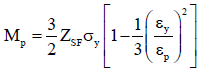(3)

Mp: plastic moment, εy: yield strain

εp: the strain when strain hardening begin

From the test result explained in the chapter 4, double type of SFD can be divided into two pieces of basic type of SFD as shown in the Figure 11 and restoring force characteristics can be expressed by sum of shear force of them. So, the skeleton curve of double type of SFD is expressed by sum of each tri-linear curve and defined at four break points. The skeleton curve of cross type of SFD is approximated as trilinear curve which is defined by the same method of basic type of SFD. Figure 12 shows the resistant mechanism of cross type of SFD. From this model, the rigidity KSF and yield strength Py of cross type of SFD are obtained theoretically as follows: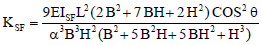(4)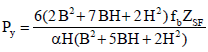(5)

From the test result explained in the chapter 4, restoring force characteristics of HSFD can be expressed by sum of shear force of double type and cross type of SFD. So, the skeleton curve of HSFD is expressed by sum of each skeleton curve and defined at six break points. Figure 12 shows the comparison of test and analytical skeleton curve and it is confirmed that the above analytical skeleton curve can chase test results approximately.

Restoring force characteristics model of HSFD

Herein, the hysteresis behavior model is assumed with the strain hardening model  as shown in the Figure 13. In this paper, the analytical coefficient β related to hardening rule is decided to correspond with test result of SFD (β=0.5). Herein, the first rigidity, second rigidity and the value of break point are determined based on the skeleton curve of HSFD as shown in the Figure 13. Figure 14 shows the comparison of test and analytical results and it is confirmed that the above analytical model can chase test results approximately.

#### Seismic Response Analysis

Herein, the restoring force characteristics models of various type of SFD were studied. So then, the seismic response analysis was performed to compare the number of necessary damper by the difference in kind of SFD installed to frame. The analytical frame model is presented on Figure 15, which is called as “trim-model”  designed with thin section member. The properties of this frame model are shown on the Fig.16. And, the seismic response analysis is performed subjected to wave of JMA-Kobe NS phase which is artificial seismic waves. The damping effect coefficients Dh are summarized on Figure 16. Herein, in this paper, the strain hardening model of hysteresis rules as shown in Figure 17. And, to control the story drift angle to less than about 1/150 rad, proper numerical SFD are arranged on the frame model. Figure 18 and Table 3 shows the distribution of maximum story drift, details of SFD installed to frame and the number of damper. From the Figure 18, it can be said that it is possible to reduce the number of dumper by use of double type of SFD or HSFD.

#### Conclusion

This paper proposed the innovated vibration control device called as scaling frame. And, to investigate the inelastic behavior and restoring force characteristics of HSFD, horizontally loading test was conducted. And also, the analytical study was done by use of restoring force characteristics model of HSFD. The remarkable

Conclusions of this paper are as follows:

1. The hysteresis behavior of HSFD shows stable during the cyclic loading.

2. The restoring force characteristics of HSFD can be expressed in the sum of shear force of double type and cross type of SFD.

3. The skeleton curve of HSFD is presented theoretically, and it shows agreements with test result approximately.

4. The hysteresis model during cyclic loading is presented by using strain hardening model, and it shows agreements with test results approximately.

5. By use of various type of SFD in substitution for basic type of SFD, it is possible to decrease the number of necessary device more.

#### References

Select your language of interest to view the total content in your interested language

### Article Usage

• Total views: 10155
• [From(publication date):
specialissue-2016 - Aug 19, 2019]
• Breakdown by view type
• HTML page views : 10078
• PDF downloads : 77

## Post your commentCan't read the image? click here to refresh
###### Peer Reviewed Journals

Make the best use of Scientific Research and information from our 700 + peer reviewed, Open Access Journals

International Conferences 2019-20

Meet Inspiring Speakers and Experts at our 3000+ Global Annual Meetings

Top